# 【C++ 继承 | 虚函数表 02】C++虚函数表剖析 ②

## 多重继承（无虚函数覆盖）

class Base1
{
public:
virtual void f() { cout << "Base1::f" << endl; }  //虚函数定义
virtual void g() { cout << "Base1::g" << endl; }
virtual void h() { cout << "Base1::h" << endl; }
};

class Base2
{
public:
virtual void f() { cout << "Base2::f" << endl; }  //虚函数定义
virtual void g() { cout << "Base2::g" << endl; }
virtual void h() { cout << "Base2::h" << endl; }
};

class Base3
{
public:
virtual void f() { cout << "Base3::f" << endl; }
virtual void g() { cout << "Base3::g" << endl; }
virtual void h() { cout << "Base3::h" << endl; }
};

class Derive :public Base1, public Base2, public Base3 //多继承的情况——无虚继承覆盖
{
public:
virtual void f1() { cout << "Derive::f1" << endl; } //虚函数定义
virtual void g1() { cout << "Derive::g1" << endl; }
};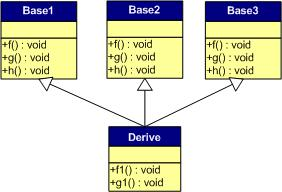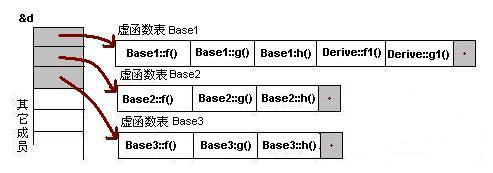1） 每个父类都有自己的虚表。
2） 子类的成员函数被放到了第一个父类的表中。（所谓的第一个父类是按照声明顺序来判断的）

## 多重继承（有虚函数覆盖）class Base1 {
public:
int ibase1;
Base1() :ibase1(10) {}
virtual void f() { cout << "Base1::f()" << endl; }
virtual void g() { cout << "Base1::g()" << endl; }
virtual void h() { cout << "Base1::h()" << endl; }

};

class Base2 {
public:
int ibase2;
Base2() :ibase2(20) {}
virtual void f() { cout << "Base2::f()" << endl; }
virtual void g() { cout << "Base2::g()" << endl; }
virtual void h() { cout << "Base2::h()" << endl; }
};

class Base3 {
public:
int ibase3;
Base3() :ibase3(30) {}
virtual void f() { cout << "Base3::f()" << endl; }
virtual void g() { cout << "Base3::g()" << endl; }
virtual void h() { cout << "Base3::h()" << endl; }
};

class Derive : public Base1, public Base2, public Base3 {
public:
int iderive;
Derive() :iderive(100) {}
virtual void f() { cout << "Derive::f()" << endl; }
virtual void g1() { cout << "Derive::g1()" << endl; }
};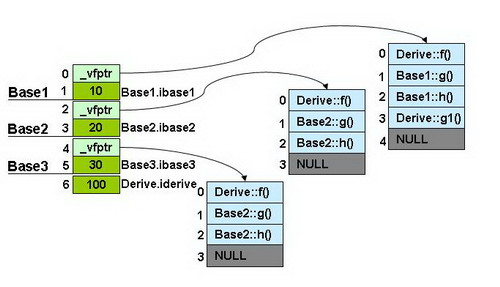• 每个父类都有自己的虚表。
• 子类的成员函数被放到了第一个父类的表中。
• 内存布局中，其父类布局依次按声明顺序排列。
• 每个父类的虚表中的f()函数都被overwrite成了子类的f()。这样做就是为了解决不同的父类类型的指针指向同一个子类实例，而能够调用到实际的函数。

## 砖石型-重复继承

class B
{
public:
int ib;
char cb;
public:
B() :ib(0), cb('B') {}
virtual void f() { cout << "B::f()" << endl; }
virtual void Bf() { cout << "B::Bf()" << endl; }
};

class B1 : public B
{
public:
int ib1;
char cb1;
public:
B1() :ib1(11), cb1('1') {}
virtual void f() { cout << "B1::f()" << endl; }
virtual void f1() { cout << "B1::f1()" << endl; }
virtual void Bf1() { cout << "B1::Bf1()" << endl; }
};

class B2 : public B
{
public:
int ib2;
char cb2;
public:
B2() :ib2(12), cb2('2') {}
virtual void f() { cout << "B2::f()" << endl; }
virtual void f2() { cout << "B2::f2()" << endl; }
virtual void Bf2() { cout << "B2::Bf2()" << endl; }
};

class D : public B1, public B2
{
public:
int id;
char cd;
public:
D() :id(100), cd('D') {}
virtual void f() { cout << "D::f()" << endl; }
virtual void f1() { cout << "D::f1()" << endl; }
virtual void f2() { cout << "D::f2()" << endl; }
virtual void Df() { cout << "D::Df()" << endl; }
};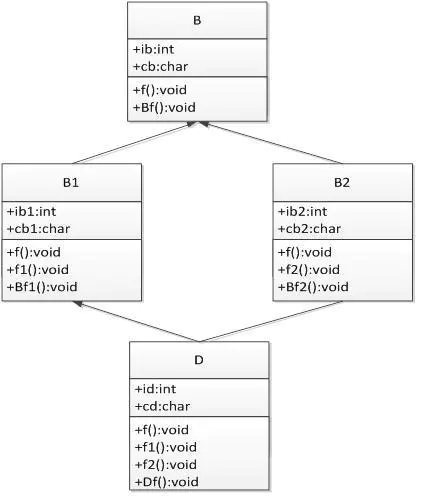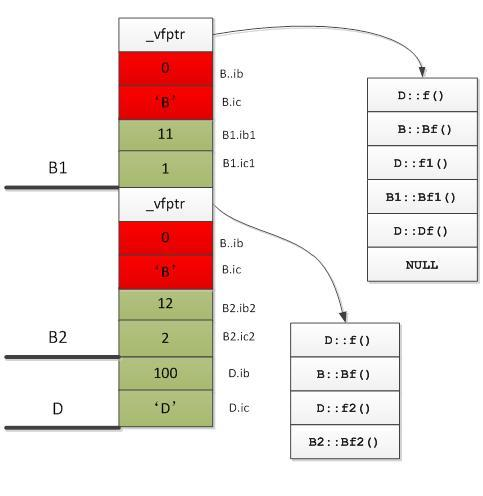1 2 3 4 D d; d.ib = 0;   //二义性错误 d.B1::ib = 1;   //正确 d.B2::ib = 2;   //正确

## 多重继承（有虚函数覆盖）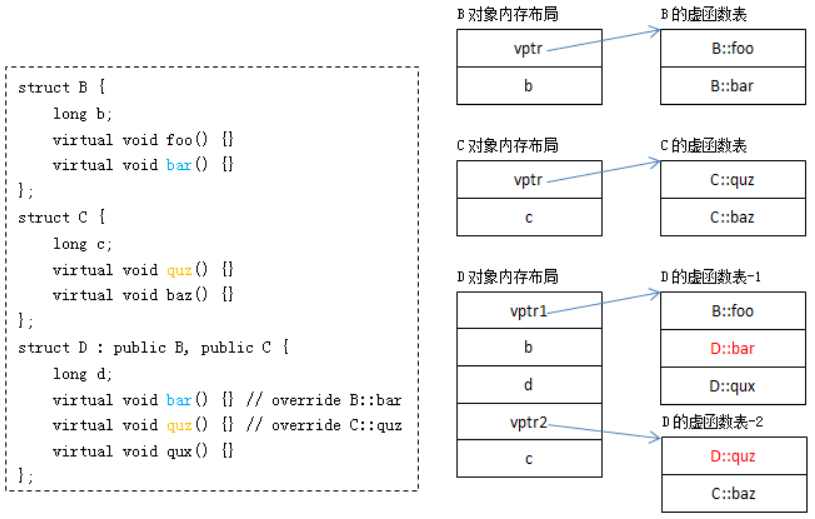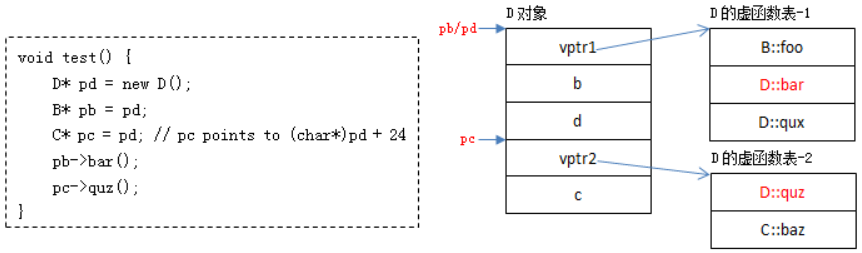## 参开资料

1. 2.5 虚函数的实现及基本原理

posted @ 2022-06-05 10:28  苏格拉底的落泪  阅读(8)  评论(0编辑  收藏  举报